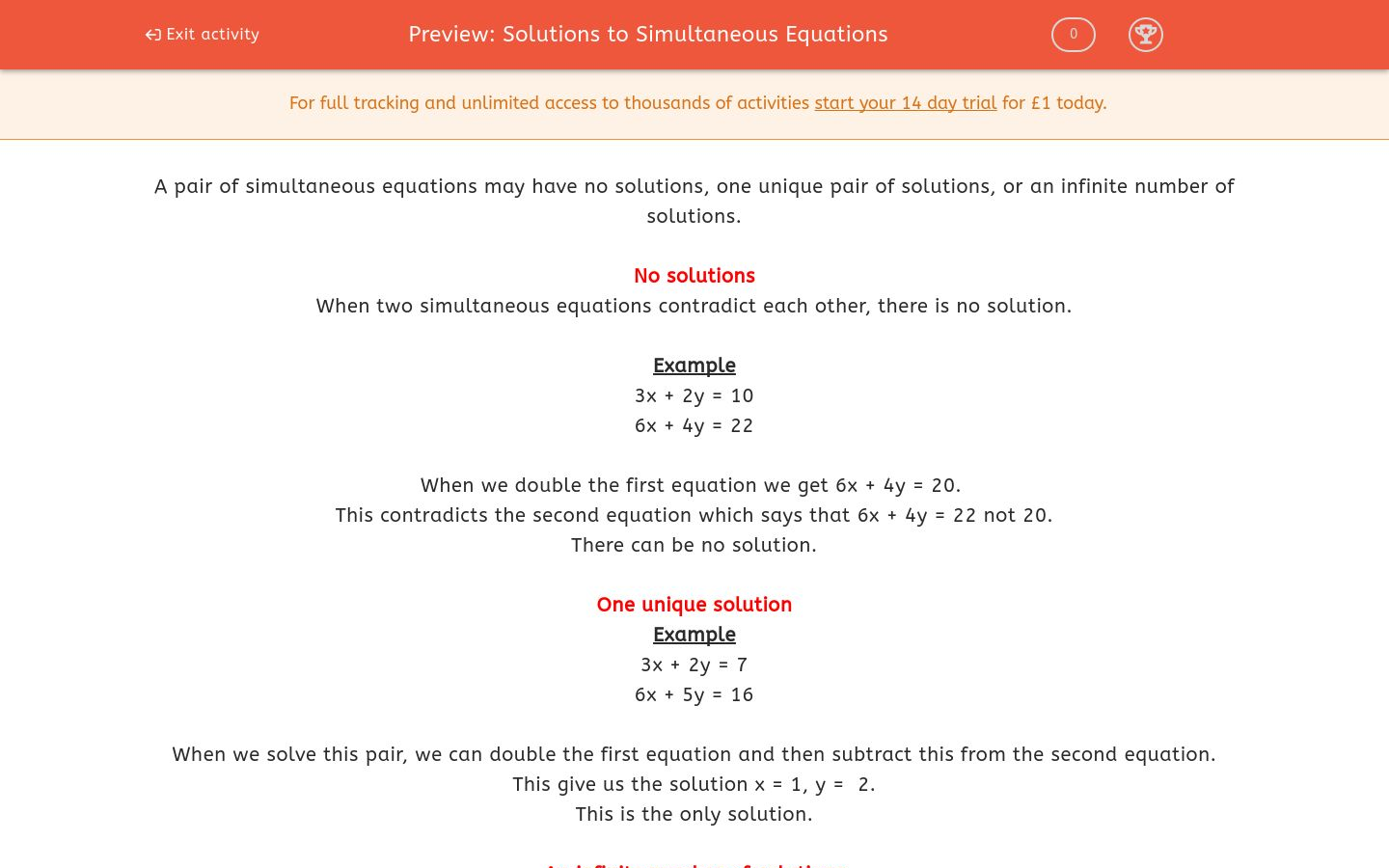# Solutions to Simultaneous Equations

In this worksheet, students state how many solutions exist to a pair of simultaneous equations.Key stage:  KS 4

Curriculum topic:  Algebra

Curriculum subtopic:  Solve Simultaneous Equations in 2 Variables

Difficulty level:### QUESTION 1 of 10

A pair of simultaneous equations may have no solutions, one unique pair of solutions, or an infinite number of solutions.

No solutions

When two simultaneous equations contradict each other, there is no solution.

Example

3x + 2y = 10

6x + 4y = 22

When we double the first equation we get 6x + 4y = 20.

This contradicts the second equation which says that 6x + 4y = 22 not 20.

There can be no solution.

One unique solution

Example

3x + 2y = 7

6x + 5y = 16

When we solve this pair, we can double the first equation and then subtract this from the second equation.

This give us the solution x = 1, y =  2.

This is the only solution.

An infinite number of solutions

When two simultaneous equations are essentially the same as each other, there are an infinite number of solutions.

Example

3x + 2y = 10

6x + 4y = 20

When we double the first equation we get the second equation.  So really we only have one equation, to which there are an infinite number of solutions.

If x = 0, y = 5.

If x = 2, y = 2.

If x = -5, y = 12.5.

Whatever value is chosen for x, you can always find a value for y that works.

Determine how many solutions there will be to the following pair of simultaneous equations:

3x + 2y = 15

4x - 3y = 20

No solutions

One unique solution

Infinite number of solutions

Determine how many solutions there will be to the following pair of simultaneous equations:

3x + 2y = 15

6x + 4y = 20

No solutions

One unique solution

Infinite number of solutions

Determine how many solutions there will be to the following pair of simultaneous equations:

3x + 2y = 15

6x + 4y = 30

No solutions

One unique solution

Infinite number of solutions

Determine how many solutions there will be to the following pair of simultaneous equations:

3x + 2y = 15

6x - 4y = 30

No solutions

One unique solution

Infinite number of solutions

Determine how many solutions there will be to the following pair of simultaneous equations:

3x + 2y = 15

2x + 3y = 15

No solutions

One unique solution

Infinite number of solutions

Determine how many solutions there will be to the following pair of simultaneous equations:

3x - 2y = 15

2y - 3x = -15

No solutions

One unique solution

Infinite number of solutions

Determine how many solutions there will be to the following pair of simultaneous equations:

3x - 2y = 15

2y - 3x = -25

No solutions

One unique solution

Infinite number of solutions

Determine how many solutions there will be to the following pair of simultaneous equations:

y = 15 - x

y = 15 - 2x

No solutions

One unique solution

Infinite number of solutions

Determine how many solutions there will be to the following pair of simultaneous equations:

y = 15 - x

y = 16 - x

No solutions

One unique solution

Infinite number of solutions

Determine how many solutions there will be to the following pair of simultaneous equations:

3y = 15 - x

3x + 9y = 45

No solutions

One unique solution

Infinite number of solutions

• Question 1

Determine how many solutions there will be to the following pair of simultaneous equations:

3x + 2y = 15

4x - 3y = 20

One unique solution
• Question 2

Determine how many solutions there will be to the following pair of simultaneous equations:

3x + 2y = 15

6x + 4y = 20

No solutions
• Question 3

Determine how many solutions there will be to the following pair of simultaneous equations:

3x + 2y = 15

6x + 4y = 30

Infinite number of solutions
• Question 4

Determine how many solutions there will be to the following pair of simultaneous equations:

3x + 2y = 15

6x - 4y = 30

One unique solution
• Question 5

Determine how many solutions there will be to the following pair of simultaneous equations:

3x + 2y = 15

2x + 3y = 15

One unique solution
• Question 6

Determine how many solutions there will be to the following pair of simultaneous equations:

3x - 2y = 15

2y - 3x = -15

Infinite number of solutions
• Question 7

Determine how many solutions there will be to the following pair of simultaneous equations:

3x - 2y = 15

2y - 3x = -25

No solutions
• Question 8

Determine how many solutions there will be to the following pair of simultaneous equations:

y = 15 - x

y = 15 - 2x

One unique solution
• Question 9

Determine how many solutions there will be to the following pair of simultaneous equations:

y = 15 - x

y = 16 - x

No solutions
• Question 10

Determine how many solutions there will be to the following pair of simultaneous equations:

3y = 15 - x

3x + 9y = 45